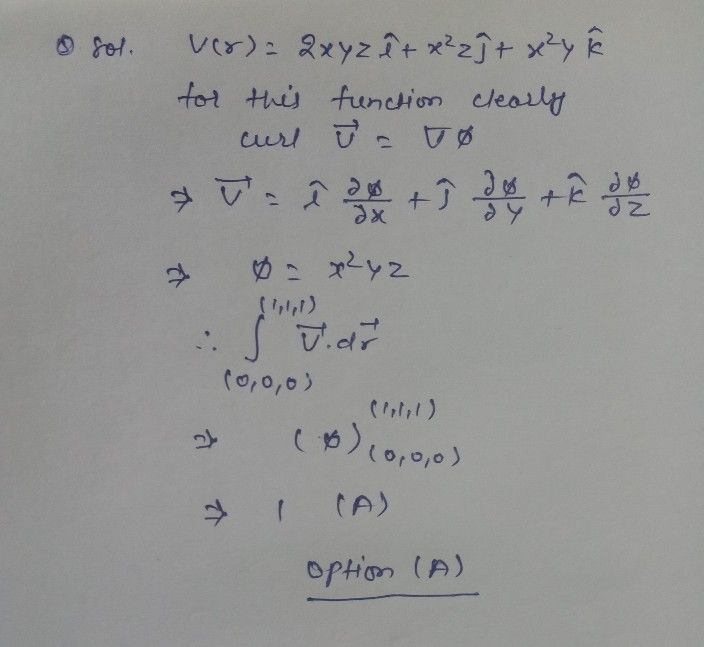Symbol
Problem$3.3$ The line integral $\int ^{\vec{V} }.$ V.dr of the vector $\vec{V} \left(\vec{i} \right)=2xy2i+x^{2}zi+x^{2}$ x²yk from the origin to the point $P\left(1,$ $1,1\right)$ (a) $\right)$ is $1$ $\left($ (b) is $2$ zero $\left($ (c) $2\right)$ $1s-1$ $\left(d\right)$ cannot be determined without specifying the $3$
Calculus
Search count: 107
SolutionQanda teacher - SNEHADear Surendra, If you have any doubt you may ask me here